Test: The Continuous, Time Fourier Series & The Discrete, Time Fourier Transform

# Test: The Continuous, Time Fourier Series & The Discrete, Time Fourier Transform - Electrical Engineering (EE)

Test Description

## 15 Questions MCQ Test GATE Electrical Engineering (EE) 2024 Mock Test Series - Test: The Continuous, Time Fourier Series & The Discrete, Time Fourier Transform

Test: The Continuous, Time Fourier Series & The Discrete, Time Fourier Transform for Electrical Engineering (EE) 2023 is part of GATE Electrical Engineering (EE) 2024 Mock Test Series preparation. The Test: The Continuous, Time Fourier Series & The Discrete, Time Fourier Transform questions and answers have been prepared according to the Electrical Engineering (EE) exam syllabus.The Test: The Continuous, Time Fourier Series & The Discrete, Time Fourier Transform MCQs are made for Electrical Engineering (EE) 2023 Exam. Find important definitions, questions, notes, meanings, examples, exercises, MCQs and online tests for Test: The Continuous, Time Fourier Series & The Discrete, Time Fourier Transform below.
Solutions of Test: The Continuous, Time Fourier Series & The Discrete, Time Fourier Transform questions in English are available as part of our GATE Electrical Engineering (EE) 2024 Mock Test Series for Electrical Engineering (EE) & Test: The Continuous, Time Fourier Series & The Discrete, Time Fourier Transform solutions in Hindi for GATE Electrical Engineering (EE) 2024 Mock Test Series course. Download more important topics, notes, lectures and mock test series for Electrical Engineering (EE) Exam by signing up for free. Attempt Test: The Continuous, Time Fourier Series & The Discrete, Time Fourier Transform | 15 questions in 45 minutes | Mock test for Electrical Engineering (EE) preparation | Free important questions MCQ to study GATE Electrical Engineering (EE) 2024 Mock Test Series for Electrical Engineering (EE) Exam | Download free PDF with solutions
 1 Crore+ students have signed up on EduRev. Have you?
Test: The Continuous, Time Fourier Series & The Discrete, Time Fourier Transform - Question 1

### Determine the Fourier series coefficient for given periodic signal x(t). Que: x(t) as shown in fig.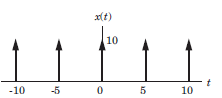​

Detailed Solution for Test: The Continuous, Time Fourier Series & The Discrete, Time Fourier Transform - Question 1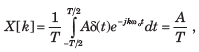A = 10 , T = 5, X [k] = 2

Test: The Continuous, Time Fourier Series & The Discrete, Time Fourier Transform - Question 2

### Determine the Fourier series coefficient for given periodic signal x(t). Que: x(t) as shown in fig.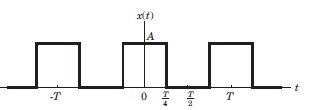​ ​ ​

Detailed Solution for Test: The Continuous, Time Fourier Series & The Discrete, Time Fourier Transform - Question 2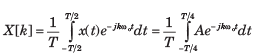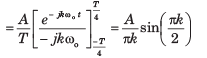Test: The Continuous, Time Fourier Series & The Discrete, Time Fourier Transform - Question 3

### Determine the Fourier series coefficient for given periodic signal x(t). Que: x(t) as shown in fig.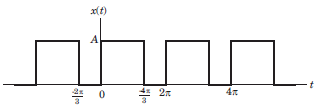​ ​ ​

Detailed Solution for Test: The Continuous, Time Fourier Series & The Discrete, Time Fourier Transform - Question 3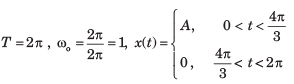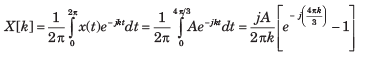Test: The Continuous, Time Fourier Series & The Discrete, Time Fourier Transform - Question 4

Determine the Fourier series coefficient for given periodic signal x(t).

Que: x(t) as shown in fig.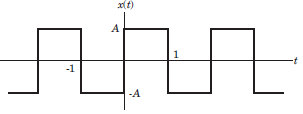​ ​ ​

Detailed Solution for Test: The Continuous, Time Fourier Series & The Discrete, Time Fourier Transform - Question 4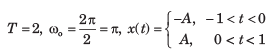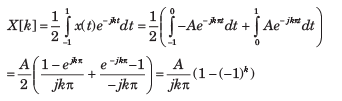Test: The Continuous, Time Fourier Series & The Discrete, Time Fourier Transform - Question 5

Determine the Fourier series coefficient for given periodic signal x(t).

x(t) = sin2t

Detailed Solution for Test: The Continuous, Time Fourier Series & The Discrete, Time Fourier Transform - Question 5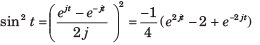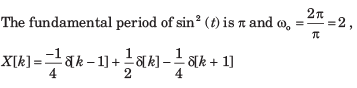Test: The Continuous, Time Fourier Series & The Discrete, Time Fourier Transform - Question 6

In the question, the FS coefficient of time-domain signal have been given. Determine the corresponding time domain signal and choose correct option.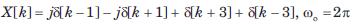Detailed Solution for Test: The Continuous, Time Fourier Series & The Discrete, Time Fourier Transform - Question 6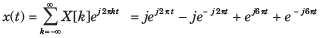= 2(cos 6πt - sin 2πt)

Test: The Continuous, Time Fourier Series & The Discrete, Time Fourier Transform - Question 7

In the question, the FS coefficient of time-domain signal have been given. Determine the corresponding time domain signal and choose correct option.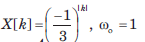Detailed Solution for Test: The Continuous, Time Fourier Series & The Discrete, Time Fourier Transform - Question 7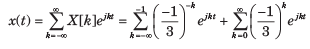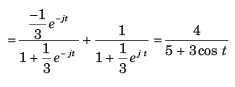Test: The Continuous, Time Fourier Series & The Discrete, Time Fourier Transform - Question 8

In the question, the FS coefficient of time-domain signal have been given. Determine the corresponding time domain signal and choose correct option.

Que: X[k] as shown in fig, wo  = π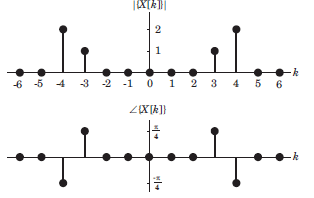Detailed Solution for Test: The Continuous, Time Fourier Series & The Discrete, Time Fourier Transform - Question 8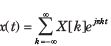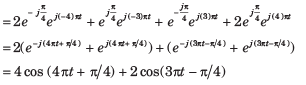Test: The Continuous, Time Fourier Series & The Discrete, Time Fourier Transform - Question 9

In the question, the FS coefficient of time-domain signal have been given. Determine the corresponding time domain signal and choose correct option.

Que: X[k] As shown in fig. , ωo = 2π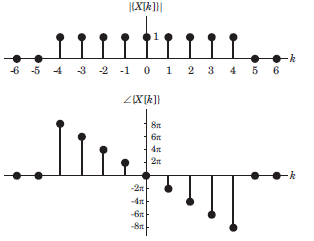Detailed Solution for Test: The Continuous, Time Fourier Series & The Discrete, Time Fourier Transform - Question 9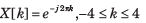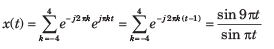Test: The Continuous, Time Fourier Series & The Discrete, Time Fourier Transform - Question 10

In the question, the FS coefficient of time-domain signal have been given. Determine the corresponding time domain signal and choose correct option.

Que: X[k] As shown in fig. , ωo = π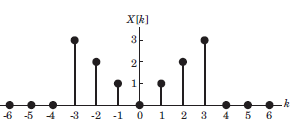Detailed Solution for Test: The Continuous, Time Fourier Series & The Discrete, Time Fourier Transform - Question 10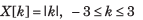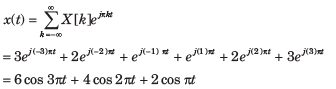Test: The Continuous, Time Fourier Series & The Discrete, Time Fourier Transform - Question 11

Consider a continuous time periodic signal x(t) with fundamental period T and Fourier series coefficients X[k]. Determine the Fourier series coefficient of the signal y(t) given in question and choose correct option.

Que: y(t) = x(t - t0 ) + x (t - t0 )

Detailed Solution for Test: The Continuous, Time Fourier Series & The Discrete, Time Fourier Transform - Question 11

x(t - t0) is also periodic with T.  TheFourier series coefficients X1[k] of x(t - t0) are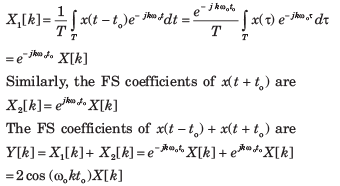Test: The Continuous, Time Fourier Series & The Discrete, Time Fourier Transform - Question 12

Consider a continuous time periodic signal x(t) with fundamental period T and Fourier series coefficients X[k]. Determine the Fourier series coefficient of the signal y(t) given in question and choose correct option.

Que: y(t) = Ev{x(t)}

Detailed Solution for Test: The Continuous, Time Fourier Series & The Discrete, Time Fourier Transform - Question 12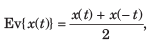The FS coefficients of x(t) are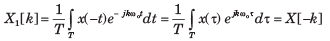Therefore, the FS coefficients of Ev{ x(t)} are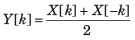Test: The Continuous, Time Fourier Series & The Discrete, Time Fourier Transform - Question 13

Consider a continuous time periodic signal x(t) with fundamental period T and Fourier series coefficients X[k]. Determine the Fourier series coefficient of the signal y(t) given in question and choose correct option.

Que: y(t) = Re{x(t)}

Detailed Solution for Test: The Continuous, Time Fourier Series & The Discrete, Time Fourier Transform - Question 13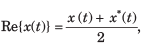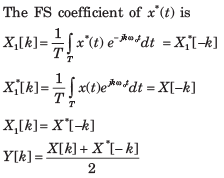Test: The Continuous, Time Fourier Series & The Discrete, Time Fourier Transform - Question 14

Determine the signal having the Fourier transform given in question.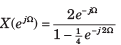Detailed Solution for Test: The Continuous, Time Fourier Series & The Discrete, Time Fourier Transform - Question 14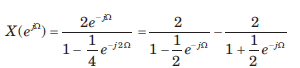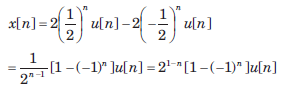Test: The Continuous, Time Fourier Series & The Discrete, Time Fourier Transform - Question 15

Determine the signal having the Fourier transform given in question.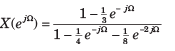Detailed Solution for Test: The Continuous, Time Fourier Series & The Discrete, Time Fourier Transform - Question 15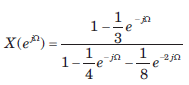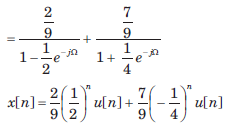## GATE Electrical Engineering (EE) 2024 Mock Test Series

23 docs|285 tests
Information about Test: The Continuous, Time Fourier Series & The Discrete, Time Fourier Transform Page
In this test you can find the Exam questions for Test: The Continuous, Time Fourier Series & The Discrete, Time Fourier Transform solved & explained in the simplest way possible. Besides giving Questions and answers for Test: The Continuous, Time Fourier Series & The Discrete, Time Fourier Transform, EduRev gives you an ample number of Online tests for practice

## GATE Electrical Engineering (EE) 2024 Mock Test Series

23 docs|285 tests